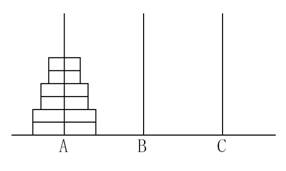# P1096 Hanoi 双塔问题

• 5.6K通过
• 15.1K提交
• 题目提供者 CCF_NOI
• 评测方式 云端评测
• 标签 数论,数学 递归 递推 高精 NOIp普及组 2007
• 难度 普及/提高-
• 时空限制 1000ms / 128MB
• 提示：收藏到任务计划后，可在首页查看。

## 题目描述

给定$A$、$B$、$C$三根足够长的细柱，在$A$柱上放有$2n$个中间有孔的圆盘，共有$n$个不同的尺寸，每个尺寸都有两个相同的圆盘，注意这两个圆盘是不加区分的（下图为$n=3$的情形）。现要将这些圆盘移到$C$柱上，在移动过程中可放在$B$柱上暂存。要求：

（1）每次只能移动一个圆盘；

（2）$A$、$B$、$C$三根细柱上的圆盘都要保持上小下大的顺序；

任务：设$A_n$为$2n$个圆盘完成上述任务所需的最少移动次数，对于输入的$n$，输出$A_n$。

## 输入输出格式

输入格式：

一个正整数$n$，表示在$A$柱上放有$2n$个圆盘。

输出格式：

一个正整数, 为完成上述任务所需的最少移动次数$A_n$。

## 输入输出样例

输入样例#1： 复制
【输入样例1】
1
【输入样例2】
2
输出样例#1： 复制
【输出样例1】
2
【输出样例2】
6

## 说明

【限制】

对于$50\%$的数据，$1 \le n \le 25$

对于$100\%$的数据，$1 \le n \le 200$

【提示】

设法建立$A_n$与$A_{n-1}$的递推关系式。

提示
标程仅供做题后或实在无思路时参考。
请自觉、自律地使用该功能并请对自己的学习负责。
如果发现恶意抄袭标程，将按照I类违反进行处理。